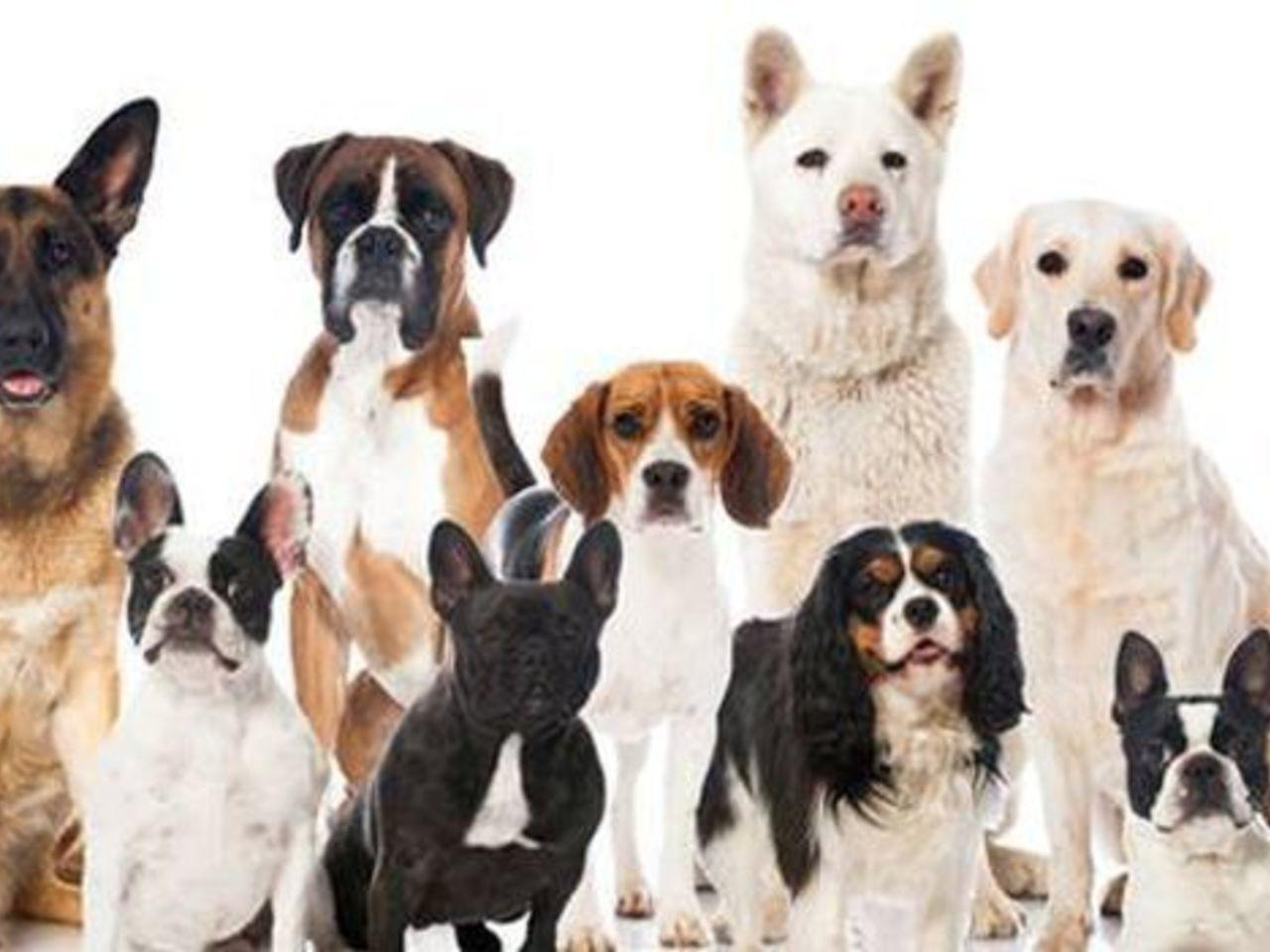# Dog Breed Classification using Transfer Learning in TensorFlowIn this article, we will go through the TensorFlow to build a Convolutional Neural Network that will help you to identify the breed of a dog from its image. In the process, we will use Transfer Learning and learn how to design, train and test models with the provided dataset.

In this article, we will go through the TensorFlow to build a Convolutional Neural Network that will help you to identify the breed of a dog from its image.  In the process, we will use Transfer Learning and learn how to design, train and test models with the provided dataset.

The whole article is divided into the following sections:

1. Dataset
2. Imports
3. Model
4. Data Processing
5. Training
6. Testing

# Dataset

For this task we are going to use the Dog Breed Identification dataset which can be easily downloaded from the Kaggle datasets. The dataset is designed for multiclass classification problem as it has 120 breeds of dogs. It provides you with the train and test images along with the labels of training images in the labels.csv file.

File descriptions

• train.zip – the training set, you are provided the breed for these dogs.
• test.zip – the test set, you must predict the probability of each breed for each image.
• sample_submission.csv – a sample submission file in the correct format.
• labels.csv – the breeds for the images in the train set.

The labels.csv file has two columns: id and breed. The id column contains the name of the images, which is used to find the breed name of the training images.

## Imports

Here we import all the packages needed for this task.

```import os
import numpy as np
import pandas as pd
import cv2
from glob import glob
import tensorflow as tf
from tensorflow.keras.layers import *
from tensorflow.keras.applications import MobileNetV2
from tensorflow.keras.callbacks import *
from keras import backend as K
from sklearn.model_selection import train_test_split```

## Model

As we have already mentioned that this task is going to use Transfer Learning. So let’s learn about Transfer learning. It is a technique of reusing a pre-trained model on a new task and here the task a multiclass classification.

```def build_model(size, num_classes=120, trainable=False):
inputs = Input((size, size, 3))
backbone = MobileNetV2(
input_tensor=inputs,
include_top=False,
weights="imagenet")
backbone.trainable = trainable
x = backbone.output

x = GlobalAveragePooling2D()(x)
x = Dropout(0.2)(x)
x = Dense(1024, activation="relu")(x)
x = Dense(num_classes, activation="softmax")(x)

model = tf.keras.Model(inputs, x)
return model```

Here we use MobileNev2 as the pre-trained model. You can also use other models like Resnet50, VGG16, VGG19 and more available in TensorFlow. We add a few layers to pre-trained model to make it ready for the classification task.

```def read_image(path, size):
image = cv2.resize(image, (size, size))
image = image / 255.0
image = image.astype(np.float32)
return image```

## Data Processing

Now we will perform pre-processing on the dataset we are using for this task. In the process , we will first create a function that is going to read the image from the path and also resize it to the desired size. Then, we perform normalization to make the image pixels smaller by dividing them with the 255.

Now we will use TensorFlow tf.data functions to create the dataset pipeline for training.

The tf_parse function preprocess the single instance of the complete dataset. It preprocesses a single image and its label and return it to the dataset function.

```def tf_parse(x, y):
def _prase(x, y):
x = x.decode()

num_classes = 120
size = 224
label =  * num_classes
label[y] = 1
label = np.array(label, dtype=np.int32)
return image, label

return tf.numpy_function(_prase, [x, y], [tf.float32, tf.int32])

def tf_dataset(x, y, batch=8):
dataset = tf.data.Dataset.from_tensor_slices((x, y))
dataset = dataset.map(tf_parse)
dataset = dataset.batch(batch)
return dataset```

# Training

Till now we have built the model and the training dataset pipeline. Now we are ready the training the model on the actual dataset.

First we set the desired path for the training images and the labels csv file with contain the name of the breeds.

```path = "Dog Breed Identification/"
train_path = os.path.join(path, "train/*")
labels_path = os.path.join(path, "labels.csv")```

Here we read the labels.csv file and create a list of the breeds of dogs used in this dataset. Then we create a dictionary which maps the name of the breed with an index number. This index number is used to uniquely identify each breed.

```labels_df = pd.read_csv(labels_path)
breed = labels_df["breed"].unique()
print("Number of Breeds: ", len(breed))
breed2id = {name:i for i, name in enumerate(breed)}```

Now we load all the training images and its respective labels from the labels.csv.

```images = glob(train_path)
labels = []
for image_path in images:
image_id = image_path.split("/")[-1].split(".")
breed_name = list(labels_df[labels_df.id == image_id]["breed"])
breed_idx = breed2id[breed_name]
labels.append(breed_idx)```

Now we split the dataset in the training and validation set by 80/20 ratio. Just make sure that train_test_split uses the same randon_state value, as it is used to seed the randomness.

```train_x, valid_x = train_test_split(images, test_size=0.2, random_state=42)
train_y, valid_y = train_test_split(labels, test_size=0.2, random_state=42)```

Here we have defined some hyperparameters that we are going to use during the training

```size = 224
num_classes = len(breed)
lr = 1e-4
batch = 16
epochs = 5```

Here we build the model and compile it by specifying the loss, optimizer and metrics used during the training.

```model = build_model(size, num_classes=num_classes, trainable=False)

Here we used the tf_dataset function to create the training and validation dataset used in the training.

```train_dataset = tf_dataset(train_x, train_y)
valid_dataset = tf_dataset(valid_x, valid_y)  ```

Here we specify two callbacks: ModelCheckpoint and ReduceLrOnPlateau.

``` callbacks = [
ModelCheckpoint("model.h5", verbose=1),
ReduceLROnPlateau(factor=0.1, patience=5, min_lr=1e-6)
]```

And finally we start the training of the model. I have just trained it on the 5 epochs, so the accuracy of the model would not be good. So you train it on more epochs to get good results.

`model.fit(train_dataset, validation_data=valid_dataset, epochs=epochs, callbacks=callbacks)`